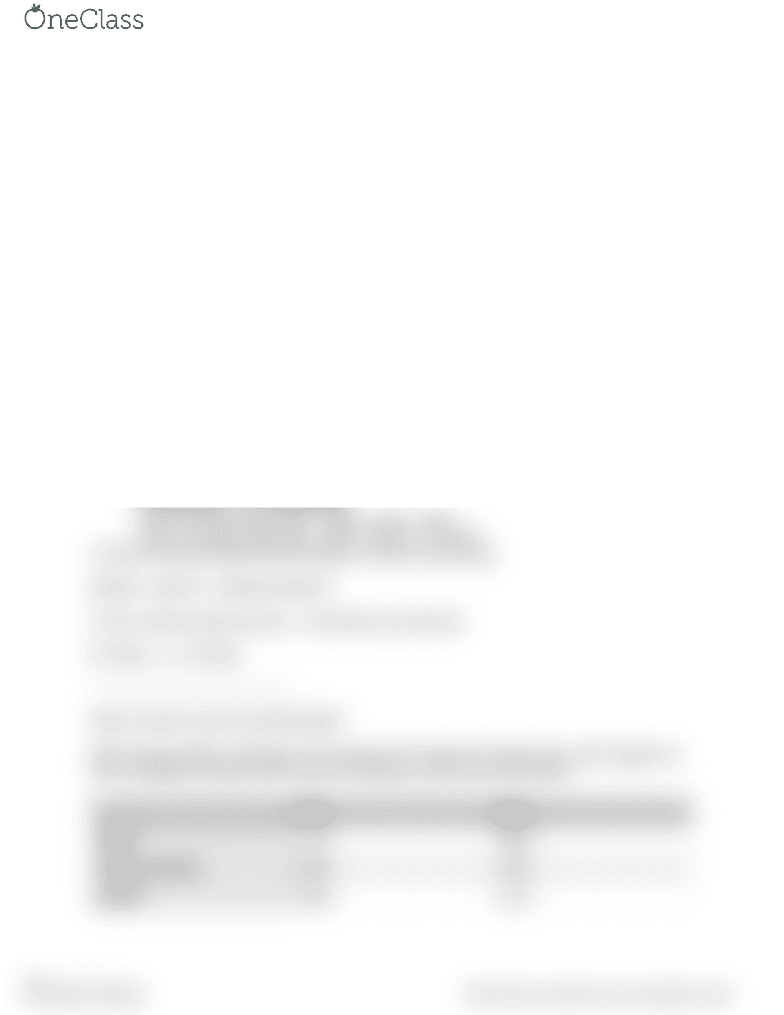Class Notes (1,100,000)
CA (650,000)
McMaster (50,000)
COMMERCE (2,000)
Lecture 20

# COMMERCE 1AA3 Lecture 20: Class 20

Department
Commerce
Course Code
COMMERCE 1AA3
Professor
Lecture
20

This preview shows half of the first page. to view the full 2 pages of the document.Financial Accounting - COMMERCE 1A03: Class 20
Check out the illustrations of cost ﬂow assumptions document.
***LOOK AT THE SLIDE ABOUT ABC INC***
For the Perpetual WAC, you must calculate the COGS for each sale.
1/7— COGS: 8000 * \$5.50
Units Available for Sale = 8000
Cost/Unit = 44000/8000 = \$5.50
COGS = 4000 * \$5.50 = \$22000
Cost of Ending Inventory after sale: 8000 - 4000 = 4000 * \$5.50 = \$22000
4/6— Cost of Inventory after last sale + Purchases since last sale
4000 * \$5.50 + 2000 * \$12.00 + 6000 * \$14.00 = 130000
Units Available for Sale = 4000 + 2000 + 6000 = 12000
Cost/Unit = 130000/12000 = \$10.83
COGS = 3000 * \$10.83 = \$32490
Units of Ending Inventory after sale = 12000 - 3000 = 9000
Cost of Ending Inventory after Sale = 9000 * \$10.83 = \$97470
9/14— Cost of Inventory after Last Sale + Purchases = 9000 * \$10.83 + 6000 * \$15
Units Available for Sale = 9000 + 6000 = 15000
Cost/Unit = 187470/15000 = \$12.50
COGS = 6000 * \$12.50 = \$75000
Units of Inventory after sale - 15000 - 6000 = 9000
Cost of Inventory after Sale - 9000 * \$12.50 = \$112500
COGS for the year (add up all the totals for COGS for each sale)
\$22000 + \$32470 + \$75000 \$1299470
Cost of Inventory after last sale + Purchases since last sale
\$112500 + 0 = \$112500
——————————————
When inventory costs are INCREASING:
!
WAC will give higher COGS than FIFO because FIFO takes the oldest units, which happens to
be the cheapest, whereas WAC takes the average of both new and old items.
FIFO
WAC
COGS
Lower
Higher
Ending Inventory
Higher
Lower
Income
Higher
Lower
find more resources at oneclass.com
find more resources at oneclass.com